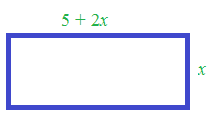### Home > AC > Chapter 4 > Lesson 4.1.3 > Problem4-24

4-24.

The length of a rectangle is five centimeters more than twice its width. The perimeter is $100$ centimeters. Use Guess and Check to find out how long and how wide the rectangle is.

Draw a diagram.

Use a guess and check table to solve this problem.GUESS Width

Length (2x + 5)

CHECK Perimeter = 100

$\$

$\$